U.S. Department of Transportation
1200 New Jersey Avenue, SE
Washington, DC 20590
202-366-4000

Federal Highway Administration Research and Technology
Coordinating, Developing, and Delivering Highway Transportation InnovationsThis report is an archived publication and may contain dated technical, contact, and link information
 Federal Highway Administration > Publications > Research Publications > Hydraulics Publications > 07026 > Bottomless Culvert Scour Study: Phase II Laboratory Report
 Publication Number: FHWA-HRT-07-026 Date: February 2007

# Bottomless Culvert Scour Study: Phase II Laboratory Report

### Chapter 6: Scour Calculation Examples

This section gives step-by-step instructions for calculating the maximum scour depth for unsubmerged bottomless culverts. Two different scenarios from the results section will be shown.

#### USING kS AS A FUNCTION OF VRA, VCL, AND F1

The first example is based on using VRA, VCL, and F1. The procedure is as follows:

Step 1: Compute the representative velocity of the flow using the average velocity in the approach section (equation 2) as follows.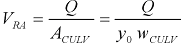(28)

where:

Q
is volumetric flow through the culvert (m3/s).
y0
is depth of flow in the approach to the culvert before scour (m).
wCULV
is width of the culvert inlet (m).

Step 2: Express the critical velocity computed by Laursen’s method (equation 5) in terms of y2 as follows.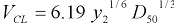(29)

where:

y2
is equilibrium flow depth (m).
D50
is sediment size (m).

Step 3: Everything in the previous two equations should be known except for y2. Now we can substitute the previous two equations into equation 1 as follows.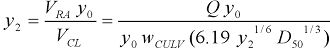(30)

This expression can now be rearranged to calculate y2 as follows.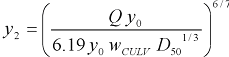(31)

Step 4: Now use the scour equations from the first entry (ks) in table 2 to calculate the maximum scour, recalling that only the intercept of these equations should be used.

Without wingwalls, the maximum scour is computed with the following equation.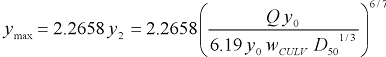(32)

Alternatively, the equation for the maximum scour with wingwalls is as follows.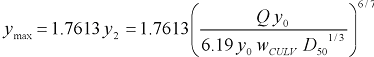(33)

#### USING ks AS A FUNCTION OF VRM, VCN, AND Qblocked

The second example is based on using VRM, VCN, and Qblocked. The procedure is as follows:

Step 1: Compute representative velocity of the flow using the calibrated velocity in the culvert inlet (equation 22) as follows.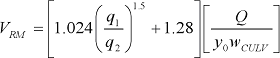(34)

where:

Q
is volumetric flow through the culvert (ft3/s or m3/s).
y0
is depth of flow in the approach to the culvert before scour (ft or m).
wCULV
is width of the culvert inlet (ft or m).
q1
is unit discharge in the approach section (ft2/s or m2/s).
q2
is unit discharge in the contracted section (ft2/s or m2/s).

Note that the unit discharge ratio of q1 divided by q2 can be computed from a width ratio as follows.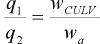(35)

where:

wCULV
is width of the bottomless culvert inlet (m).
wa
is width of the approach section to the culvert (m).

Step 2: Express the critical velocity computed by Neill’s method (equations 6, 7, and 8, or 9) in terms of y2. For example, for D50 sediment size greater than 0.0003 m (0.001 ft) but less than 0.03 m (0.1 ft), the equation for Neill’s critical velocity is given as follows.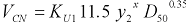(36)

The exponent, x, is calculated using equation 37: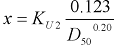(37)

where:

y2
is equilibrium flow depth, m or ft.
D50
is sediment size, m or ft.
KU1
is 0.3048(0.65-x) for SI units, or 1.0 for U.S. customary units.
x
is the exponent from equation 8.
KU2
is 0.788 for SI units, or 1.0 for U.S. customary units.

Step 3: Everything in the previous three equations should be known except for y2. Now we can substitute the previous two equations into equation 1 as follows.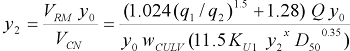(38)

This expression can now be rearranged to calculate y2 as follows.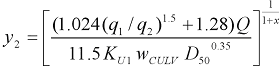(39)

Step 4: Now use the scour equations from the first entry (ks) in table 2 to calculate the maximum scour.

Without wingwalls, the maximum scour is computed with the following equation.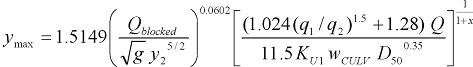(40)

Alternatively, the equation for the maximum scour with wingwalls is as follows.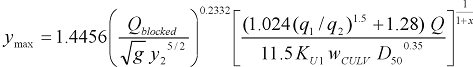(41)

Previous | Contents | Next